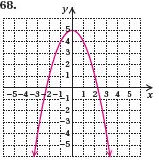# Finding parabola equation and only given a graph

## Homework Statement

The math problem I am supposed to do is, find an equation for the given graph.

## Homework Equations

There are no equations given, but here's an image for it.## The Attempt at a Solution

I understand there is an equation like
y=ax^2+bx+c but I'm not sure how I can apply it to this graph. I know the Vertex of the Parabola is (0,5) and the line of symmetry I think it's called is x=0, but what I'm confused at is what the actual equation would be.

If anyone can give me a clue as how I can solve it, that would be great!

Kevin_Axion
Put it in vertex form $$y = a(x + h)^2 + k$$ where h is the x value of the vertex and k is the y value. The answer should then be $$y = ax^2 + 5$$, you can solve for a by plugging in values of x, y and then isolate a, there is a clear one $$(1, 4)$$. I don't know if I can give this much information though.

Especially at 1 AM!